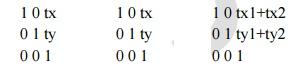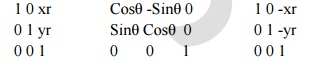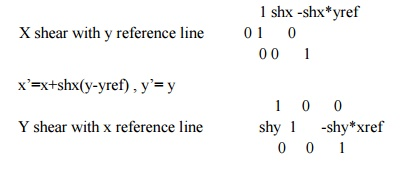Home | | Graphics and Multimedia | Two Dimensional Graphics Transformations

# Two Dimensional Graphics Transformations

Geometric Transformations: Changes in size, shape are accomplished with geometric transformation. It alter the coordinate descriptions of object.

TWO DIMENSIONAL GRAPHICS TRANSFORMATIONS

Geometric Transformations

Changes in size, shape are accomplished with geometric transformation. It alter the coordinate descriptions of object.

The basic transformations are Translation, Roatation, Scaling. Other transformations are Reflection and shear.Basic transformations used to reposition and resize the two dimentional objects.

Two Dimensional Transformations

Translation

A Translation is applied to an object by repositioning it along a straight line path from one co-ordinate location to another. We translate a two dimensional point by adding translation distances tx and ty to the original position (x,y) to move the point to a new location (x’,y’)

X’=x+tx Y’=y+ty

triangle = { p1=(1,0), p2=(2,0), p3=(1.5,2) }It moves objects without deformation. (ie) Every point on the objet is translated by the same amount. It can be applied to lines, polygons.

Rotation

A two dimensional rotation is applied to an object by repositioning it along a circular path in the xy plane. To generate a rotation, we specify a rotation angle theta and the position (xr,yr) of the rotation point ( or pivot point) about which the object is to be rotated.

Positive value of the rotation angle defines counter clock wise rotation. Negative value of the rotation angle defines the clock wise rotation.

X’=xcosθ – y sinθ

Y’=xsinθ + y cosθ

Using column vector P’=P*R  R= Cosθ -Sinθ

Sinθ CosθRotation of an arbitary pivot point

Rotation of a point about any specified rotation position (xr,yr)

X’= Xr +(X-Xr)Cosθ –(Y-Yr)Sinθ

Y’=Yr+(X-Xr)Sinθ +(Y-Yr)Cosθ

It moves objects without deformations. Every point on an object is rotated through the same angle.

Scaling

A scaling transformation alters the size of an object. This operation can be carried out for polygon by multiplying the coordinate values (x,y) of each vertex by scaling factors sx and sy to produce the transformed coordinates (x’,y’).

X’=x.sx

Y’=y.sy

P= X1 P’= X1’ S= sx 0

X2     X2’    0 sy

P’=P*S

If sx=sy , then it produces the uniform scaling

Sx<> sy , different scaling.

If sx,sy<0, then it produces the reduced object size

If sx,sy > 0, then it produces the enlarged size objects.By choosing the position called fixed point, we can control the location of the scaled object. This point is remain unchanged after the scaling transformation.

X’= Xf +(X-Xf)sx => X’= X.sx +(Xf(1-sx))

Y’=Yf+(Y-Yf)sy => Y’= Y.sy +Yf(1-sy)

Matrix representations and homogeneous coordinates

Graphics applications involves sequences of geometric transformations. The basic transformations expressed in terms of

P’=M1 *P +M2

P, P’ à Column vectors.

M1 à 2 x 2 array containing multiplicative factors

M2 à 2 Element column matrix containing translation terms

For translation à M1 is the identity matrix

For rotation or scaling àM2 contains transnational terms associated with the pivot point or scaling fixed point.

For coordinate positions are scaled, then rotated then translated, these steps are combined together into one step, final coordinate positions are obtained directly from the initial coordinate values.

To do this expand the 2 x 2 matrix into 3 x 3 matrix.

To express 2 dimensional transformation as a multiplication, we represent each cartesion coordinate position (x,y) with the homogeneous co ordinate triple (Xh,Yh, h) where

X= xh/h, Y=Yh/h

So we can write (h.x, h.y,h), set h=1. Each two dimensional position is represented with homogeneous coordinates(x,y,1). Coordinates are represented with three element column vector. Transformation operations are written as 3 by 3 matrices.

For translationP’=T(tx,ty)*P

Inverse of the translation matrix is obtained by replacing tx, ty by –tx, -tyP’= R(θ)*P

We get the inverse rotation matrix when θ is replaced with (-θ)P’= S(sx,sy)*P

Composite transformations

Sequence of transformations is called as composite transformation. It is obtained by forming products of transformation matrices is referred as a concatenation (or) composition of matrices.

Translation: -

Two successive translationsT(tx1,ty1) + T(tx2,ty2) = T(tx1+tx2, ty1+ty2)

Rotation

R(θ1)* R(θ2)= R(θ1+ θ2)

P’=P. R(θ1+ θ2)

ScalingS(x1,y1).S(x2,y2) = S(sx1.sx2 , sy1.sy2)

1.  the order we perform multiple transforms can matter

·        eg. translate + scale can differ from scale + translate

·        eg. rotate + translate can differ from translate + rotate

·        eg. rotate + scale can differ from scale + rotate (when scale_x differs from scale_y)

2.  When does M1 + M2 = M2 + M1?General pivot point rotation

Rotation about any selected pivot point (xr,yr) by performing the following sequence of translate

– rotate – translate operations.

1. Translate the object so that the pivot point is at the co-ordinate origin.

2. Rotate the object about the coordinate origin

3. Translate the object so that the pivot point is returned to its original positionConcatenation properties

T(xr,yr).R(θ).T(-xr,-yr) = R(xr,yr, θ)

Matrix multiplication is associative. Transformation products may not be commutative.

Combination of translations, roatations, and scaling can be expressed as

X’ rSxx      rSxy trSx             X

Y’ rSyx      rSyy trSy    Y

1        0        0        1        1

Other transformations

Besides basic transformations other transformations are reflection and shearing

Reflection :

Reflection is a transformation that produces the mirror image of an object relative to an axis of reflection. The mirror image is generated relative to an axis of reflection by rotating the object by 180 degree about the axis.

Reflection about the line y=0 (ie about the x axis), the x-axis is accomplished with the transformation matrix.

1 0 0

0 -1 0

0 0 1

It keeps the x values same and flips the y values of the coordinate positions. Reflection about the y-axis

-1 0 0 0 1 0 0 0 1

It keeps the y values same and flips the x values of the coordinate positions. Reflection relative to the coordinate origin.

-1 0 0 0 -1 0 0 0 1

Reflection relative to the diagonal line y=x , the matrix is 0 1 0 1 0 0 0 0 1

Reflection relative to the diagonal line y=x , the matrix is 0 -1 0 -1 0 0 0 0 1

Shear

A transformation that alter the shape of an object is called the shear transformation. Two shearing transformations

1. Shift x coordinate values ( X- shear)

2. Shifts y coordinate values. (Y-shear)

In both cases only one coordinate ( x or y ) changes its coordinates and other preserves its values.

X –Shear

It preserves the y value and changes the x value which causes vertical lines to tilt right or leftX’= X+shx*y

Y’=Y

Y –Shear

It preserves the x value and changes the y value which causes vertical lines to tilt right or left 1 shy 0

Y-sh = 0 1 0 0 0 1

Y’= Y+shy*X

X’=XShearing Relative to other reference line

We can apply x and y shear transformations relative to other reference lines. In x shear transformation we can use y reference line and in y shear we can use x reference line.

The transformation matrices for both are given below.which generates transformed coordinate positions. x’=x , y’= shy(x-xref)+y

This transformation shifts a coordinate position vertically by an amount proposal to its distance from the reference line x=x ref.

Transformations between coordinate systems

Transformations between Cartesian coordinate systems are achieved with a sequence of translate-rotate transformations. One way to specify a new coordinate reference frame is to give the position of the new coordinate origin and the direction of the new y-axis. The direction of the new x-axis is then obtained by rotating the y direction vector 90 degree clockwise. The transformation matrix can be calculated as the concatenation of the translation that moves the new origin to the old co-ordinate origin and a rotation to align the two sets of axes. The rotation matrix is obtained from unit vectors in the x and y directions for the new system

Study Material, Lecturing Notes, Assignment, Reference, Wiki description explanation, brief detail
Computer Graphics and Multimedia : Two Dimensional Graphics Transformations |